﻿ quartz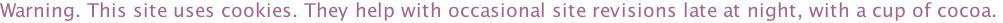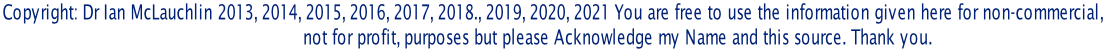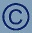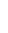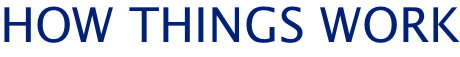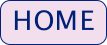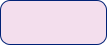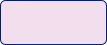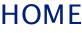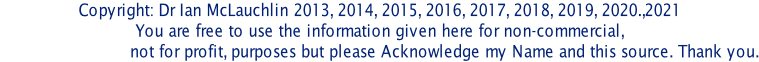TIME AND HOW A QUARTZ CLOCK WORKSA clock tells the time. Let’s look at that a bit more closely. A clock ‘knows’ the time and then it tells us what that time is. But what is time?

And what does ‘telling it to us’ mean?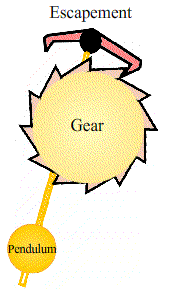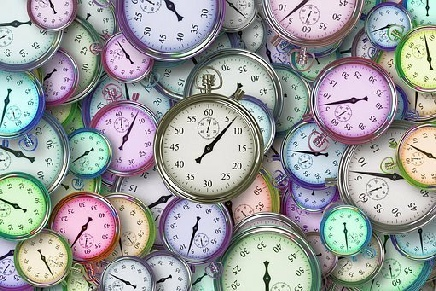In order to tell us, a clock must be able to measure it. Humans have worked out that seasons correspond to the stages of the earth moving round the sun and that days are related to the spin of the earth as it travels. It takes a year for the earth to make one trip around the sun. That’s also 12 lunar cycles. Those are months. One day equals the time taken for the earth to spin a complete revolution about its own axis. So we can observe how long a day is. We went to bed when it got dark and got up when it was light again. For most of history that's all we needed to know about time.But what if we want to measure less than a day? We need to divide a day up somehow.

The ancient Babylonians used a base system of 60 and divided the circle into 360 parts. The ancient Greeks divided the earth into 360 imaginary lines of longitude and latitude. That would mean that the passage of time, ie the rate of rotation of the earth, could be measured in terms of lines of longitude. But how we divided the duration of a day up is complicated.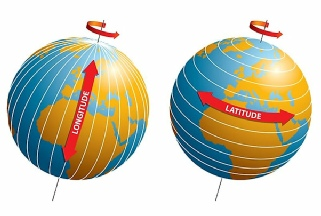Eventually it was decided that there would be 24 hours (2 x 12) in a day and the ‘Babylonian 60’ divided that into sixty small units and those units into 60 too. So an hour was 60 minutes and a minute was 60 seconds.

So that’s a brief history of time! But how do we measure it?We need to find something that can count time. We can do it out loud – as a speaking clock - so ‘1 and 2 and 3 and etc’ roughly counts seconds but we wouldn’t inflict that on anyone. What we need is a physical property that depends on time. Luckily for us there are a few.

The earliest clocks used a swinging pendulum. A weight on the end of a long rod always takes the same time to swing backwards and forwards. We can devise a mechanism of cogs to count the number of swings and connected hands to show the advance of time. If the pendulum is the right length it will swing once every second. Trouble is, air resistance causes it to slow down and eventually stop. So we need some way of keeping the pendulum swinging.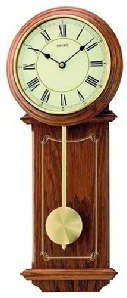Church clocks use a hanging weight which gives the pendulum a boost at every swing by means of an escapement which stops the mechanisms from spinning and lets the gears move in time with the pendulum. Unfortunately, the weight has to be wound back up the tower, usually every day.